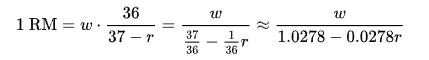×
EVEN THE mini TOOLS CAN EMPOWER PEOPLE TO DO GREAT THINGS.

# One Rep Max (1RM) Calculator

 Weight Lifted: kg lb Number of Repetitions: 1 2 3 4 5 6 7 8 9 10 11 12 13 14 15 16 17 18 19 20

## About One Rep Max (1RM) Calculator

The One Rep Max (1RM) Calculator is used to calculate your one-rep maximum (one repetition maximum or 1RM), which is the maximum amount of weight one can lift in a single repetition for a given exercise.

There are several common formulas used to calculate 1RM using the submaximal method. Our One Rep Max (1RM) Calculator is using Brzycki Formula to calculate 1RM.Reference this content, page, or tool as:

"One Rep Max (1RM) Calculator" at https://miniwebtool.com/one-rep-max-1rm-calculator/ from miniwebtool, https://miniwebtool.com/